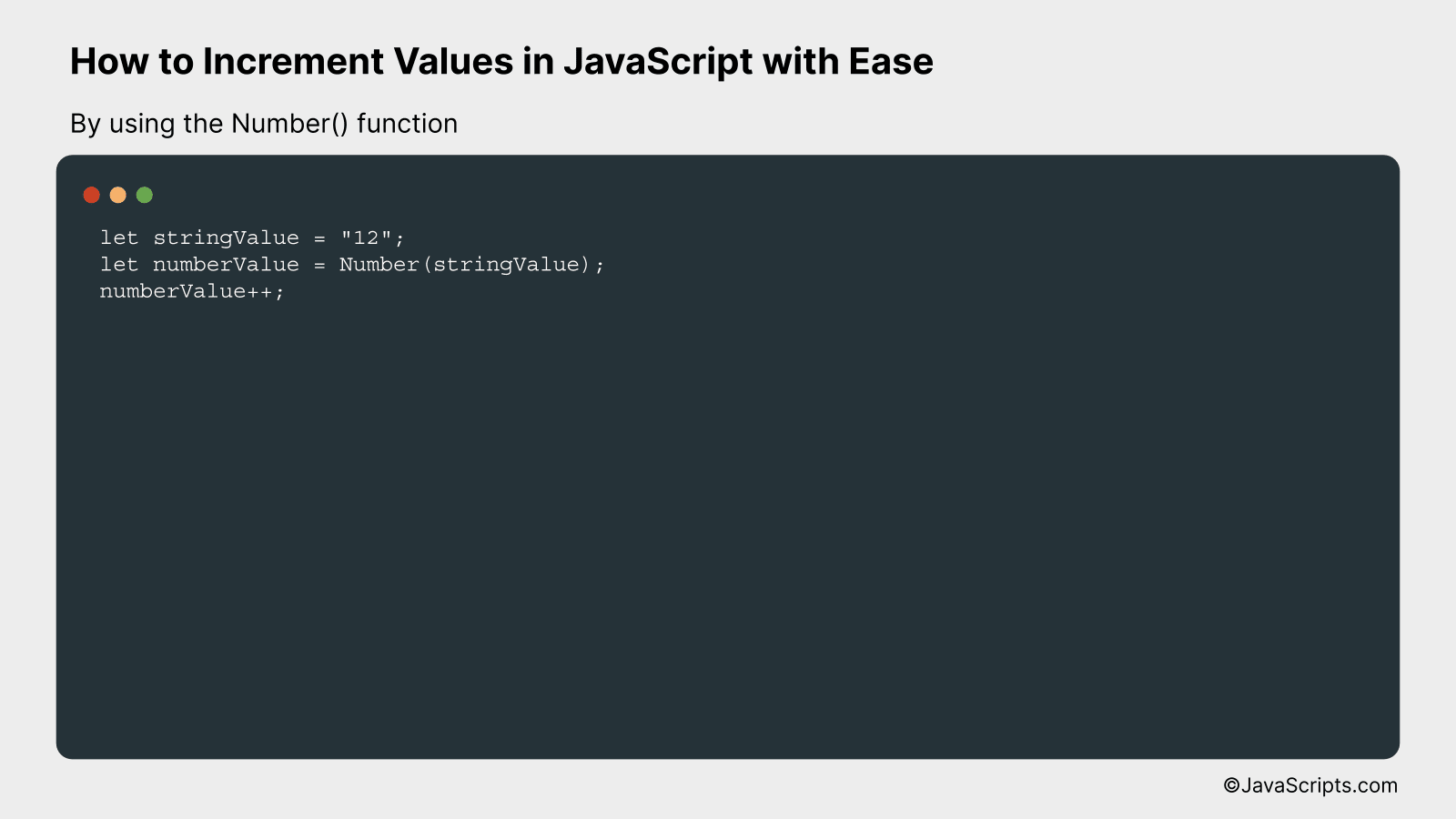# How to Increment Values in JavaScript with Ease

JavaScript is one of the most versatile and user-friendly programming languages out there. It opens the door to a myriad of possibilities, and one of those is the ability to increment values swiftly and efficiently.

Did you ever wonder how to add up numbers seamlessly in your code? We’re about to unfold an easy guide to incrementing values in JavaScript. You and I will delve into this topic together, breaking down the concepts into bite-sized, easy-to-understand pieces.

In this post, we will be looking at the following 3 ways to increment values in JavaScript with ease:

• By using the increment operator (++)
• By using the addition assignment operator (+=)
• By using the Number() function

Let’s explore each…

## #1 – By using the increment operator (++)The increment operator (++) in JavaScript is used to increase the value of a variable by 1. In this example, we’ll declare a variable with an initial value, and then increment it using ++.

``````
var count = 0;
console.log(count); // Outputs: 0
count++;
console.log(count); // Outputs: 1
```
```

### How it Works

The increment operator (++) adds 1 to the value of the variable it’s applied to. This operation can be very useful in loops, counters, and many other programming situations where a value needs to be gradually increased.

• First, we declare a variable named ‘count’ and give it an initial value of 0.
• Next, we output the value of ‘count’ to the console, which will be 0, as this is the initial value we set.
• We then use the increment operator (++) to add 1 to the value of ‘count’.
• Finally, we output the value of ‘count’ again, and this time the console will display 1, as the value has been increased by the increment operator.

## #2 – By using the addition assignment operator (+=)The JavaScript addition assignment operator (+=) simplifies the process of incrementing a variable by adding a specific value to it. For example, if you want to add 5 to a variable ‘x’, you can simply use ‘x += 5’ instead of ‘x = x + 5’.

``````
let x = 10;
x += 5;
``````

### How it works

The ‘x += 5’ is a shorthand for ‘x = x + 5’. The operator += adds the value on its right to the variable on its left and then assigns the result back to the variable.

• First, variable ‘x’ is initialized with a value ’10’.
• Then using the addition assignment operator (+=), we add ‘5’ to ‘x’. This is equivalent to ‘x = x + 5’.
• The modified value of ‘x’ is now ’15’.

## #3 – By using the Number() functionWe will be using the JavaScript Number() function to convert a string to a number and then increment it. This is done by first converting the string into a number using Number() function, then we will use the ++ operator after the variable to increase its value by one.

``````
let stringValue = "12";
let numberValue = Number(stringValue);
numberValue++;
``````

### How it works

The Number() function in JavaScript converts a string to a number, allowing it to be incremented using the ++ operator. This process is useful when handling string data that needs to be used in mathematical operations.

• First, we initialize a variable (stringValue) with a string value containing a numeric value. In the provided example, we’ve used “12”.
• Next, we use the Number() function to convert this string into a number. We store this numeric value into a new variable (numberValue).
• Lastly, we use the incremental operator (++) on the numberValue variable. This operator increases the value of numberValue by one.

Related:

In conclusion, incrementing values in JavaScript is quite straightforward. It’s as simple as using the ‘++’ operator or adding a number to the existing value with the ‘+=’ operator.

Remember, practice is key for mastering any programming concept. With regular practice, incrementing values in JavaScript will become second nature. So, keep coding and keep learning.